VIGENERE SQUARE CIPHER CODE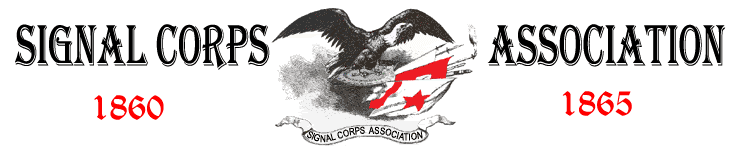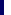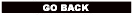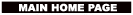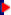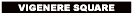The Vigenère cipher uses 26 distinct cipher alphabets!

The 26 cipher alphabets are contained in the Vigenère Square, shown below, which has a plaintext alphabet followed by 26 cipher alphabets, each one shifted by one more letter with respect to the previous one. Hence, row number 1 represents a cipher alphabet with a Caesar shift of 1, row number 2 represents a cipher alphabet with a Caesar shift of 2, and so on.

A different row of the square can be used to encrypt each letter of the message. In other words, the sender might encrypt the first letter according to row 5, the second according to row 14, and the third letter according to row 21, and so on. In order to unscramble the message, it is important that the intended receiver knows which row of the Vigenère Square has been used to encipher each letter, and so there must be an agreed system of switching between rows. This agreement is achieved via a keyword.

To illustrate how a keyword is used in conjunction with the Vigenère Square, let us encipher 'Divert Troops To East Ridge', using the keyword WHITE. First, the keyword is spelled out above the message, and repeated until each letter in the message is associated with a letter from the keyword. This can be done by clicking on the 'Repeat Keyword' button.

Above first letter of the message, 'd', is the key letter, W. To encrypt this first letter, we go to the column headed by 'd' and then see where it intersects the row starting with W, which is at the letter Z. Consequently, the letter 'd' in the plaintext is represented by Z in the ciphertext. You can check this by clicking on 'Letter Encipher'.

This process is repeated to encrypt other letters in the message. The second letter is 'i', and above it is the key letter H. To encrypt 'i', we identify the column headed by 'i' and see where it intersects the row starting with H, which is at the letter P. Consequently, the letter 'i' in the plaintext is represented by P in the ciphertext. You can check the encryption of this letter and subsequent letters by clicking on the 'Letter Encipher' button.

On our next page, you will find the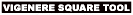which allows you to encrypt any message by choosing any keyword.

(Below is the Vigenere Square)

 Keyword: Plaintext: Ciphertext: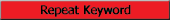A B C D E F G H I J K L M N O P Q R S T U V W X Y Z B C D E F G H I J K L M N O P Q R S T U V W X Y Z A C D E F G H I J K L M N O P Q R S T U V W X Y Z A B D E F G H I J K L M N O P Q R S T U V W X Y Z A B C E F G H I J K L M N O P Q R S T U V W X Y Z A B C D F G H I J K L M N O P Q R S T U V W X Y Z A B C D E G H I J K L M N O P Q R S T U V W Z Y Z A B C D E F H I J K L M N O P Q R S T U V W X Y Z A B C D E F G I J K L M N O P Q R S T U V W X Y Z A B C D E F G H J K L M N O P Q R S T U V W X Y Z A B C D E F G H I K L M N O P Q R S T U V W X Y Z A B C D E F G H I J L M N O P Q R S T U V W X Y Z A B C D E F G H U J K M N O P Q R S T U V W X Y Z A B C D E F G H I J K L N O P Q R S T U V W X Y Z A B C D E F G H I J K L M O P Q R S T U V W X Y Z A B C D E F G H I J K L M N P Q R S T U V W X Y Z A B C D E F G H I J K L M N O Q R S T U V W X Y Z A B C D E F G H I J K L M N O P R S T U V W X Y Z A B C D E F G H I J K L M N O P Q S T U V W X Y Z A B C D E F G H I J K L M N O P Q R T U V W X Y X A B C D E F G H I J K L M N O P Q R S U V W X Y Z A B C D E F G H I J K L M N O P Q R S T V W X Y Z A B C D E F G H I J K L M N O P Q R S T U W X Y Z A B C D E F G H I J K L M N O P Q R S T U V X Y Z A B C D E F G H I J K L M N O P Q R S T U V W Y Z A B C D E F G H I J K L M N O P Q R S T U V W X Z A B C D E F G H I J K L M N O P Q R S T U V W X Y